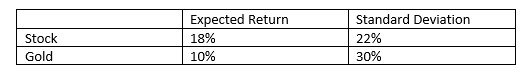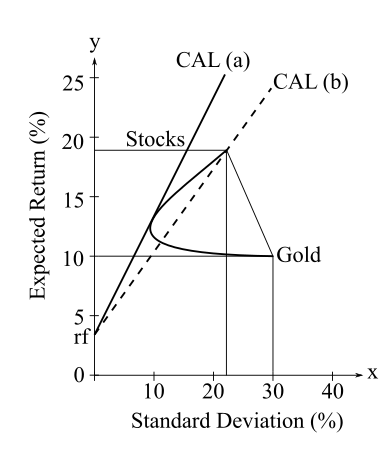# Stocks offer an expected rate of return of 18%, with a standard deviation of 22%. Gold offers an expected return of 10% with a standard deviation of 30%.a. In light of the apparent inferiority of gold with respect to both mean return and volatility, would anyone hold gold? If so, demonstrate graphically why one would do so.b. Given the data above, reanswer (a) with the additional assumption that the correlation coefficient between gold and stocks equals 1. Draw a graph illustrating why one would or would not hold gold in one’s portfolio. c. Could the set of assumptions in part (b) for expected returns, standard deviations, and correlation represent an equilibrium for the security market?

Question
40 views

Stocks offer an expected rate of return of 18%, with a standard deviation of 22%. Gold offers an expected return of 10% with a standard deviation of 30%.
a. In light of the apparent inferiority of gold with respect to both mean return and volatility, would anyone hold gold? If so, demonstrate graphically why one would do so.
b. Given the data above, reanswer (a) with the additional assumption that the correlation coefficient between gold and stocks equals 1. Draw a graph illustrating why one would or
would not hold gold in one’s portfolio.
c. Could the set of assumptions in part (b) for expected returns, standard deviations, and correlation represent an equilibrium for the security market?

check_circle

Step 1(a)

As per the given scenario, stock of 18% expected return dominates the gold of 10% expected return irrespective of that gold is an appealing asset to be held as a portfolio segment. Gold will be part of the portfolio if the correlation between stocks & gold is much low. Here, to hold gold can be an option. Gold will be part of an optimum portfolio.

As per the graph shown above, the capital asset line CAL (a) shows a steeper slope for the capital asset line for the portfolio that only consists of stock indicated by capital asset line CAL (b).

Step 2

(b)

Gold will be the part of the portfolio when the correlation of stocks & gold will be equals to 1.

As per the graph shown above, the stock and gold standard deviation (risk) and expected return combination will indicate a neg...

### Want to see the full answer?

See Solution

#### Want to see this answer and more?

Solutions are written by subject experts who are available 24/7. Questions are typically answered within 1 hour.*

See Solution
*Response times may vary by subject and question.
Tagged in